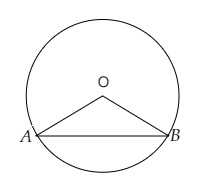# In a circle of radius $6 \mathrm{~cm}$, a chord of length $10 \mathrm{~cm}$ makes an angle of $110^{\circ}$ at the centre of the circle. Find the area of the circle.

Given:

Radius of the circle $r=6 \mathrm{~cm}$.

Length of the arc $l=10 \mathrm{~cm}$.

Angle subtended at the centre $=110^{\circ}$.

To do:

We have to find the area of the circle.

Solution:Let $OA$ and $OB$ are the radii of the circle and $AB$ the chord.

We know that,

Area of a circle of radius $r$ is $\pi r^2$.

Therefore,

Area of the circle $=3.14 \times 6 \times 6\ cm$

$=113.04\ cm^2$

The area of the circle is $113.04\ cm^2$.

Updated on: 10-Oct-2022

30 Views